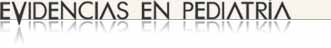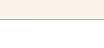# Measures of potential impact in epidemiology (1). Absolute measures of impact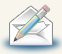Regularly recieve most recent articles by e-mail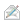Subscribe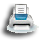Print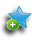Add to library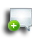Discuss this article

### MBE | Bases of evidence based medicine

Authors: Ortega Páez E1, Molina Arias M2.
2Servicio de Gastroenterología. Hospital Infantil Universitario La Paz. Madrid. España.
Correspondence: Eduardo Ortega Páez. Email: edortegap@gmail.com
Reception date: 30/11/2017
Acceptance date: 05/12/2017
Publication date: 13/12/2017

Ortega Páez E, Molina Arias M. Measures of potential impact in epidemiology (1). Absolute measures of impact. Evid Pediatr. 2017;13:62.

### MBE | Bases of evidence based medicine

Authors: Ortega Páez E1, Molina Arias M2.
2Servicio de Gastroenterología. Hospital Infantil Universitario La Paz. Madrid. España.
Correspondence: Eduardo Ortega Páez. Email: edortegap@gmail.com
Reception date: 30/11/2017
Acceptance date: 05/12/2017
Publication date: 13/12/2017

## Introduction

In epidemiology, the investigation of the association between risk factors and disease can be approached in different ways. One is to determine whether the association could or could not be due to chance with a specific level of confidence, usually 95%, using tests of statistical significance. Greater complexity and additional information can be obtained by describing the strength and direction of the association through measures of effect or association, such as the relative risk (RR)1 in cohort studies and the odds ratio (OR)2 in case-control studies. Even more information can be obtained by assessing the potential impact of removing a given risk factor on the incidence of disease in the population; these are the measures of potential impact, which can only be calculated in the context of prevention studies, such as cohort and case-control studies. Measures of potential impact could be divided into absolute measures of impact (AMIs), which measure the excess risk of exposed individuals in the reference population, and relative measures of impact (RMIs), which measure the proportion of the risk that can be attributed to exposure. If we calculate the number of individuals who are at risk or in who the event of interest occurs, we obtain potential impact numbers (PINs). In this article, we will focus on absolute measures and numbers of potential impact (aPINs).

## Absolute measures of potential impact in cohort studies

As noted above, these measure the excess risk of exposed individuals in the reference population. Their application is of great interest in health planning, as they can estimate the health benefits that could derive from the removal of the risk factor.

For the purpose of illustration, in this article we will use a fictitious cohort study analysing the association between maternal smoking and low birth weight over a 1-year period, with 230 smoking pregnant women in the exposed cohort and 320 non-smoking mothers in the unexposed cohort (Table 1).

Table 1. Absolute measures of impact and impact numbers in cohort studies . Show/hide

Attributable risk among the exposed (ARe) or attributable risk3

This measure is used when the reference population is the exposed population. It is the absolute difference in incidence between exposed individuals (Ie) and unexposed individuals (I0): ARe = Ie – I0. It measures the portion of the incidence of disease that is due to the risk factor, that is, the amount of risk in exposed individuals that is attributable solely to exposure. In our example, it would be:

$$AR_e=I_e- I_o= \frac{30}{230}- \frac{20}{320} = 0,13 - 0,062 = 0,068.$$

If we removed tobacco use in pregnant women who smoke, we would prevent low birth weight in an average of 6.8 (rounding, 7) newborns per 100 births per year.

When the incidence in the exposed population is unknown, we can estimate the ARe based on the relative risk (RR) (Table 1):

$$AR_e = I_o (RR – 1) = 0,062 (2,09 – 1) = 0,068.$$

Population attributable risk (PAR)3

Used when the reference population is the general population. It is the portion of the incidence of disease in the population that can be attributed to exposure to the risk factor in the general population. It is calculated by subtracting the incidence in the unexposed (I0) from the incidence in the total population (Ip):

$$PAR = I_p – I_o.$$

Usually, Ip is not known, and in that case it can be calculated from the Are and the proportion of exposed individuals in the target population (Pe): PAR = ARe × Pe. In our example,

$$PAR = 0,068 (\frac{230}{550}) = 0,28.$$

If we removed tobacco use in the general population, we would prevent low birth weight in approximately 3 newborns per 100 births per year.

Calculation of 95% confidence intervals

Until now, we have been making point estimates, but it is also advisable to calculate their 95% confidence intervals (95 CIs), as they will help us determine whether our results may or not be due to chance. Since we have calculated a difference in risk, the interval cannot contain the value of zero. In the case of the ARe, which is a risk difference, the 95% CI can be calculated using the Wald interval for the difference between proportions,4 substituting the upper and lower limits of the ARe 95% CI in the equation (Table 1).

## Absolute measures of potential impact in case-control studies

For the purpose of illustration, we will use a case-control study that analysed the association between infection by Helicobacter pylori (HP) and the presence of colorectal polyps in children. The study included patients with colorectal polyps and healthy controls, and the presence of HP infection was assessed in both groups5 (Table 2).

Table 2. Absolute measures of impact and impact numbers in case-control studies. Show/hide

Attributable risk among the exposed (ARe) or attributable risk3

The case-control design does not allow the direct calculation of incidences. Alternatively, it is possible to calculate the proportion of individuals exposed in the population under study (Pe) and the incidence in the unexposed (I0), assuming that the controls are representative of the population and that the incidence of disease is low (< 10%). Under these conditions, the OR can be used to approximate the RR.

The ARe is used when the reference population is the population of exposed individuals. Although it can be calculated with the same method used for cohort studies substituting the OR for the RR, this is a poor approximation (ARe = I0 – OR). The best approximation is the following:

$$AR_e = \frac{I_o (OR-1)}{P_ec (OR-1)+1} .$$

Usually, the incidence in the unexposed (I0) is not known, in which case it can be approximated by the incidence in the general population, which can be obtained from previous studies in the literature. Pec represents the proportion of controls that have been exposed in the population under study.

In our example:

$$OR = \frac{20 × 61}{6 × 15} = 4,67; CI \,95: \,1,51 \,to \,14,41.$$

Let us assume I0 = 1%. Pec = 6 / 27=0.22, so entering the values in the equation gives:

$$AR_e = \frac{0,01 (4,67-1)}{0,22 (4,67-1)+1}= 0,0203=2,02%.$$

If HP were eradicated in patients infected by HP, it would prevent an average of 202 colon polyps per 10 000 individuals.

Population attributable risk (PAR)3

It is calculated by the same method used for cohort studies, substituting Pec for Pe.

$$RAP = AR_e × P_cte = 0,020 × 0,22 = 0,0044.$$

If HP were eradicated in the general population, it would prevent an average of 44 cases of colon polyps per 10 000 individuals.

The 95 CI cannot be calculated, as the CIs for the incidence in the general population and the incidence in exposed controls are not known.

## Absolute potential impact numbers in cohort studies6

Clinicians may have difficulty understanding measures of impact that refer to individuals at risk from a general perspective, and it would be more intuitive to translate these measures to the individual level. Starting from the number needed to treat (NNT), which expresses the number of individuals that may benefit from or be harmed by a given intervention, we can derive impact numbers (INs), which refer to the number of individuals that benefit from or are harmed by exposure to a risk factor, and are given by the reciprocals of measures of impact. They provide additional information on the risks and benefits of interventions and it is possible to calculate 95 CIs for them, but they have the drawback, as does the NNT, that the 95 CI is difficult to interpret when the measures of impact they derive from are not statistically significant, which is why their use is not recommended when this is the case.

Exposure impact number (EIN)

It is the number of exposed individuals among whom one excess case is attributable to the exposure. It is given by the reciprocal of the ARe.

$$EIN = \frac{1}{AR_e} = \frac{1}{I_e – I_o}.$$

In our example:

$$EIN = \frac{1}{0,068} = 14,7 \simeq 15.$$

On average, for every 15 pregnant women who smoke, 1 will have a child with low birth weight attributable to tobacco use per year.

Population impact number (PIN)

It is the number of individuals in the whole population amongst whom one case is attributable to exposure to the risk factor. It is the reciprocal of the PAR.

$$PIN = \frac{1}{RAP} = AR_e × P_e.$$

In our example:

$$PIN = \frac{1}{0,028} = 35,7 \simeq 36.$$

On average, for every 36 pregnant women in the general population, 1 will have a child with low birth weight attributable to smoking each year.

Calculation of 95% confidence intervals7

For the EIN, the lower limit of the CI corresponds to the upper limit of the ARe CI, and the upper limit to the lower limit of the ARe CI (Table 1).

The calculation for the PIN is more complex. The PIN can be expressed based on the RR as follows:

$$PIN = \frac{1}{I_o × P_e (RR – 1)} .$$

Having done this, the 95 CI can be obtained by substituting the lower limits of the RR CI for the upper limits of the PIN CI, and vice versa (Table 1).

## Absolute potential impact numbers in case-control studies6

Exposure impact number (EIN)

In case-control studies, it is better to calculate confidence intervals based on the OR:

$$EIN = \frac{1}{I_o (OR – 1)} = 0,01 (4,67 – 1) = 27,2 \simeq 28.$$

On average, for every 28 individuals infected by HP, 1 will have colon polyps.

Population impact number (PIN)

It is calculated from the Io, the Pec and the OR.

$$PIN = \frac{1}{I_o × P_ec} × (OR – 1).$$

Substituting the values, we obtain:

$$PIN = \frac{1}{0,01} × 0,22 × (4,67 – 1) = 122,7 \simeq 123.$$

On average, for every 123 individuals in the general population there is 1 individual with colon polyps attributable to infection by HP.

Calculation of 95% confidence intervals7

The method is the same one applied in cohort studies, except that in this case it is the confidence interval of the OR that is used, substituting the upper limit of the OR CI for the lower limit of the PIN CI, and vice versa (Table 2).

Ortega Páez E, Molina Arias M. Measures of potential impact in epidemiology (1). Absolute measures of impact. Evid Pediatr. 2017;13:62.

## References

1. Molina Arias M, Ochoa Sangrador C. Estudios observacionales (II). Estudios de cohortes. Evid Pediatr. 2014;10:14.
2. Molina Arias M, Ochoa Sangrador C. Estudios observacionales (III). Estudios de casos y controles. Evid Pediatr. 2014;10:33.
3. Nieto FJ, Peruga A. Riesgo atribuible: sus formas, usos e interpretación. Gac Sanit. 1990;18:112-7.
4. Papel de la estadística. In: Argimón JM, Jiménez J. Métodos de investigación clínica y epidemiológica. Madrid: Elsevier; 2004. p. 257.
5. Cuestas Montañés E, Ortega Páez E. La presencia de Helicobacter pylori en pólipos colorrectales no garantiza asociación causal. Evid Pediatr. 2012;8:62.
6. Heller RF, Dobson AJ, Attia J, Page J. Impact numbers: measures of risk factor impact on the whole population from case-control and cohort studies. J Epidemiol Community Health. 2002;56:606-10.
7. Hildebrandt M, Bender R, Gehrmann U, Blettner M. Calculating confidence intervals for impact numbers. BMC Med Res Methodol. 2006;6:32.
13/12/2017